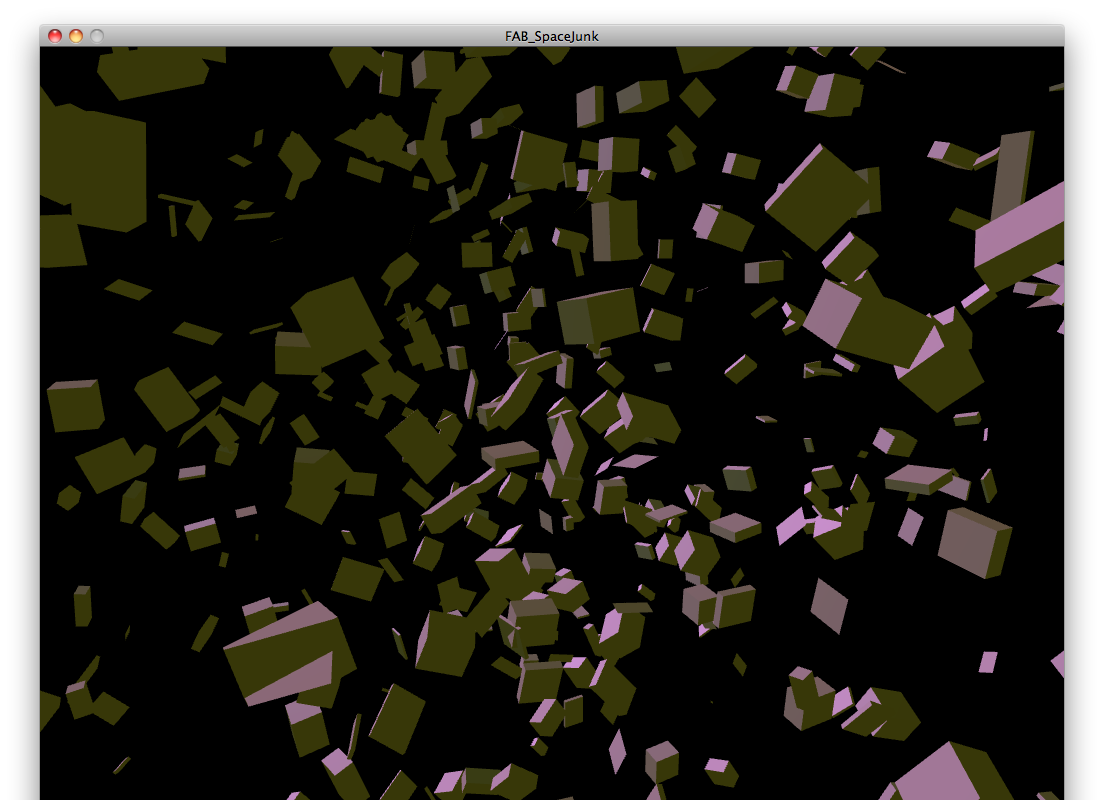# Week 12 - Application Programming

## ProcessingTo incorporate my input device into a software application, I chose to start with one of the OpenGl library examples. Starting with the example "Space Junk" (File->Examples->Libraries->OpenGL) I tailored the code to work with the serial data coming from my infrared sensor. The serial stream from the sensor sends distance interpolation which in turn reflects a zoom feature in the sketch. I took the example code I needed to add the "import processing.serial.*;" code to allow use of the serial library, and I needed to call an instance of the Serial class via the "SerialPort" variable. I replaced the data captured by the "MouseX" command and adjusted the scaling factor of the incoming data. Future developments would take this ability to visualize sensor data and incorporate both IR sensors on my input board. Being able to show the information from both sensors would allow me to triangulate physical objects. This ability to distance objects would be perfect for many robotic applications. Processing code: ``` /** * Space Junk * by Ira Greenberg. * Zoom suggestion * by Danny Greenberg. * * Rotating cubes in space using a custom Cube class. * Color controlled by light sources. Move the mouse left * and right to zoom. */ //Serial edit by Joshua Holt import processing.opengl.*; import processing.serial.*; // Used for oveall rotation float ang; Serial serialPort; // Create object from Serial class // Cube count-lower/raise to test P3D/OPENGL performance int limit = 500; // Array for all cubes Cube[]cubes = new Cube[limit]; void setup() { size(1024, 768, OPENGL); background(0); noStroke(); // Instantiate cubes, passing in random vals for size and postion for (int i = 0; i< cubes.length; i++){ cubes[i] = new Cube(int(random(-10, 10)), int(random(-10, 10)), int(random(-10, 10)), int(random(-140, 140)), int(random(-140, 140)), int(random(-140, 140))); } serialPort = new Serial(this, Serial.list(), 9600); } void draw(){ background(0); fill(200); // Set up some different colored lights pointLight(51, 102, 255, 65, 60, 100); pointLight(200, 40, 60, -65, -60, -150); // Raise overall light in scene ambientLight(70, 70, 10); while (serialPort.available () > 0) { // Center geometry in display windwow. // you can change 3rd argument ('0') // to move block group closer(+)/further(-) translate(width/2, height/2, -200 + serialPort.read()*12); // Rotate around y and x axes rotateY(radians(ang)); rotateX(radians(ang)); // Draw cubes for (int i = 0; i < cubes.length; i++){ cubes[i].drawCube(); } // Used in rotate function calls above ang++; } } ```